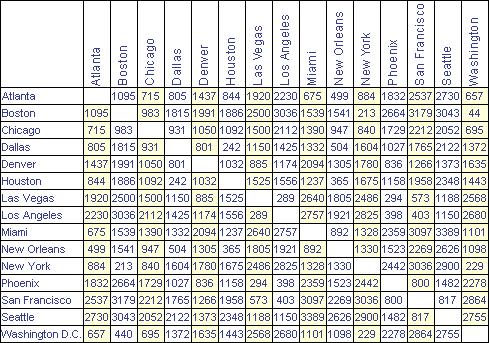# Measurement: Distance

28 teachers like this lesson
Print Lesson

## Objective

SWBAT determine the distance between points using metric units and a meter stick or metric ruler.

#### Big Idea

Students practice precise and accurate measurement of distance.

## Engage

10 minutes

Most students have practice and background knowledge surrounding the concept of distance. However, measuring distance using metric units with precision to the tenths place may or may not be within a student's wheelhouse. In fact, one of the most challenging parts of measuring distance can be the fine-motor skills and patience required to measure small distances. This lesson can be used as review or as an introductory lesson.

Making accurate and precise measurements is a student need when planning and carrying out successful investigations (SEP3). Measuring distances is not specifically stated, but it is a science practice that underpins many of the Next Generation Science Standards for Physical Science. These standards assume that students can make precise and accurate measurements in order to collect data in investigations of motion, forces, energy and even quantification of matter (since volume calculations may rely on distance measurements).

In order to ENGAGE students in this lesson, students complete a data analysis exercise. Students answer this prompts using the following chart of distances between cities.

1) What do you think this data table shows?

2) What are two conclusions you can make about the data?Students share their responses to the prompts. From this discussion, students generate the idea that this is a mileage chart showing distances between cities. By asking students what they mean by distance, the idea that distance is the separation or space between two points. When this definition surfaces, students write it down on the Measurement Distance Student Handout.

## Explore

40 minutes

The EXPLORE stage of the lesson is to get students involved in the topic so that they start to build their own understanding. To help students explore the concept of distance, students use a variety of resources in small groups to complete various activities on the Measurement Distance Student Handout. In order for these activities to go smoothly, it is important to provide resources that will help students independently find the information. Develop a resource list for each table or listed on a web-site that provides quick access to the resources available. Examples of some helpful resources are:

1) Science Textbook

5) BrainPop Movie: Measuring Matter

While students EXPLORE, they will need support accessing the resources and clarifying their understanding. During this time, rotate through groups to ask students probing questions about the concept. The questions may range in Bloom's Revised Taxonomy complexity from "remember" type questions such as: "What is the tool called we use to measure distance?" to "create" questions such as: "If you wanted to create a top ten list of the most important parts of measuring distance, what would include?"

For additional discussion about the pitfalls associated with teaching metric measurement, refer to the reflection in this section.

## Explain

10 minutes

The EXPLAIN stage provides students with an opportunity to communicate what they have learned so far and figure out what it means. During this stage of the lesson, we review the Measurement Distance Student Handout together. I model for students how to neatly complete the activity and we briefly discuss each topic as we review. This stage of the lesson presents a great place for a quick formative assessment because students are able to explain their answers and ask questions about parts of the activity they don't yet understand. Here is an example of a completed version: Measurement Distance Student Work. When we finish reviewing, students are offered a clean copy of the notes: Measurement Distance Student Handout Notes.

## Extend

The EXTEND stage allows students to apply new knowledge to a novel situation. The novel situation in this case is found at the end of the Measurement Distance Student Handout. For students who move through the activities quickly, I check their work and give them the choice to:

1) Review the concepts by making flashcards, quizzing or reading additional sections from the textbook.

2) Help another student who needs additional support. (See: Mastery Learning in Science: Students as Teachers)

3) Complete a kinesthetic extension activity that includes creating a scale drawing of the room using metric measurements.

4) Create a metric ruler tutorial video such as this one:

## Evaluate

20 minutes

The EVALUATION stage is for both students and teachers to determine how much learning and understanding has taken place. The primary way I evaluate student learning is by giving students an assessment that focuses on the "remember", "understand" and "apply" levels of understanding: Measurement Distance Checkout Quiz.

Additional discussion about what to do if students "don't get it" is included in the reflection for this section. Additional practice and re-assessment may be required. If so, here are a couple of resources:Measurement Distance Metric Ruler Practice and Measurement Distance Checkout Quiz Retake.